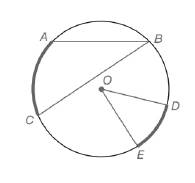Chapter 6.1, Problem 4E### Elementary Geometry for College St...

6th Edition
Daniel C. Alexander + 1 other
ISBN: 9781285195698

#### Solutions

Chapter
Section### Elementary Geometry for College St...

6th Edition
Daniel C. Alexander + 1 other
ISBN: 9781285195698
Textbook Problem
3 views

# For Exercises 1 to 8, use the figure provided.Exercises 1-8If m A C ⌢ = 56.4 ∘ ,  find m ∠ B .

To determine

To calculate:

The mB, if mAC=56.4 by using the provided figure.

Explanation

Given:

The provided figure is

Theorem used:

The measure of an inscribed angle of a circle is one-half the measure of its intercepted arc.

Calculation:

To find: mB.

Given that, mAC=56.4.

Therefore, mB is the one-half the measure of its intercepted arc.

Here the intercepted arc is AC.

### Still sussing out bartleby?

Check out a sample textbook solution.

See a sample solution

#### The Solution to Your Study Problems

Bartleby provides explanations to thousands of textbook problems written by our experts, many with advanced degrees!

Get Started

#### Convert the expressions in Exercises 6584 to power form. 23x1.2x2.13

Finite Mathematics and Applied Calculus (MindTap Course List)

#### For f(x) = 3x + 1 and g(x) = x, ?

Study Guide for Stewart's Single Variable Calculus: Early Transcendentals, 8th

#### What is the general purpose of a manipulation check?

Research Methods for the Behavioral Sciences (MindTap Course List)